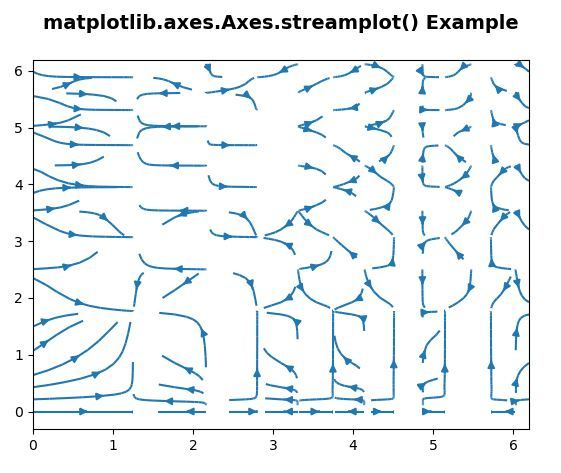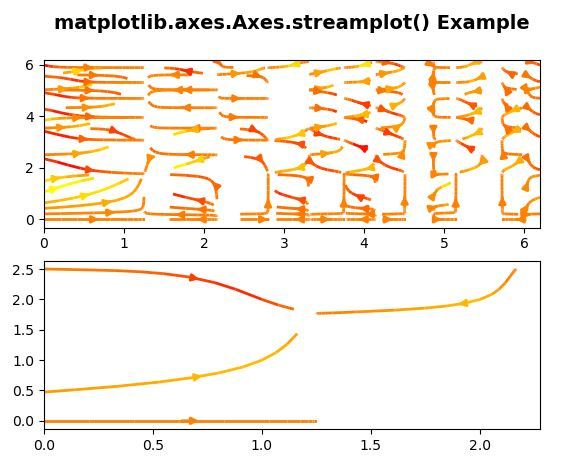# Matplotlib.axes.Axes.streamplot() in Python

Matplotlib is a library in Python and it is numerical – mathematical extension for NumPy library. The Axes Class contains most of the figure elements: Axis, Tick, Line2D, Text, Polygon, etc., and sets the coordinate system. And the instances of Axes supports callbacks through a callbacks attribute.

## matplotlib.axes.Axes.streamplot() Function

The Axes.streamplot() function in axes module of matplotlib library is also used to draw streamlines of a vector flow..

Syntax: Axes.streamplot(axes, x, y, u, v, density=1, linewidth=None, color=None, cmap=None, norm=None, arrowsize=1, arrowstyle=’-|>’, minlength=0.1, transform=None, zorder=None, start_points=None, maxlength=4.0, integration_direction=’both’, *, data=None)

Parameters: This method accept the following parameters that are described below:

• X, Y : These parameter are the x and y coordinates of the evenly spaced grid.
• U, V: This parameter is the The number of rows and columns must match the length of y and x.
• density : This parameter is used to controls the closeness of streamlines.
• linewidth : This parameter is the width of the stream lines.
• color : This parameter is the streamline color.
• cmap : This parameter is used to plot streamlines and arrows.
• norm : This parameter is used to normalize object used to scale luminance data to 0, 1.
• arrowsize : This parameter is the scaling factor for the arrow size.
• minlength : This parameter is the minimum length of streamline in axes coordinates..
• maxlength : This parameter is the maximum length of streamline in axes coordinates.
• zorder : This parameter is the zorder of the stream lines and arrows.

Returns:This method returns the following:

• stream_container :This returns the StreamplotSet
Container object with attributes

Below examples illustrate the matplotlib.axes.Axes.streamplot() function in matplotlib.axes:

Example 1:

 `# Implementation of matplotlib function ` `import` `matplotlib.pyplot as plt ` `import` `numpy as np ` `      `  `X, Y ``=` `np.meshgrid(np.arange(``0``, ``2` `*` `np.pi, .``2``), ` `                   ``np.arange(``0``, ``2` `*` `np.pi, .``2``)) ` `U ``=` `np.cos(X``*``*``2``) ` `V ``=` `np.sin(Y``*``*``2``) ` ` `  `fig, ax ``=` `plt.subplots() ` `ax.streamplot(X, Y, U, V, density ``=``[``0.5``, ``1``]) ` ` `  `ax.set_title('matplotlib.axes.Axes.streamplot()\ ` ` ``Example\n``', fontsize = 14, fontweight ='``bold') ` `plt.show() `

Output:Example 2:

 `# Implementation of matplotlib function ` ` `  ` `  `import` `matplotlib.pyplot as plt ` `import` `numpy as np ` `      `  `X, Y ``=` `np.meshgrid(np.arange(``0``, ``2` `*` `np.pi, .``2``), ` `                   ``np.arange(``0``, ``2` `*` `np.pi, .``2``)) ` `U ``=` `np.cos(X``*``*``2``) ` `V ``=` `np.sin(Y``*``*``2``) ` ` `  `fig, (ax, ax1)``=` `plt.subplots(nrows ``=` `2``, ncols ``=` `1``) ` `ax.streamplot(X, Y, U, V, density ``=``[``0.5``, ``1``], ` `             ``color ``=` `V ``*` `U, linewidth ``=` `2``, ` `             ``cmap ``=``'autumn'``) ` `val ``=` `np.array([[``2``, ``1``, ``0``, ``1``, ``2``, ``1``], ` `                ``[``2``, ``1``,  ``0``, ``1``, ``2``, ``2``]]) ` ` `  `ax1.streamplot(X, Y, U, V, color ``=` `V ``*` `U, linewidth ``=` `2``, ` `               ``cmap ``=``'autumn'``,  ` `               ``start_points ``=` `val.T) ` ` `  `ax.set_title('matplotlib.axes.Axes.streamplot() \ ` `Example\n``', fontsize = 14, fontweight ='``bold') ` `plt.show() `

Output:Attention geek! Strengthen your foundations with the Python Programming Foundation Course and learn the basics.

To begin with, your interview preparations Enhance your Data Structures concepts with the Python DS Course.

My Personal Notes arrow_drop_upSmall things always make you to think big

If you like GeeksforGeeks and would like to contribute, you can also write an article using contribute.geeksforgeeks.org or mail your article to contribute@geeksforgeeks.org. See your article appearing on the GeeksforGeeks main page and help other Geeks.

Please Improve this article if you find anything incorrect by clicking on the "Improve Article" button below.

Article Tags :

Be the First to upvote.

Please write to us at contribute@geeksforgeeks.org to report any issue with the above content.